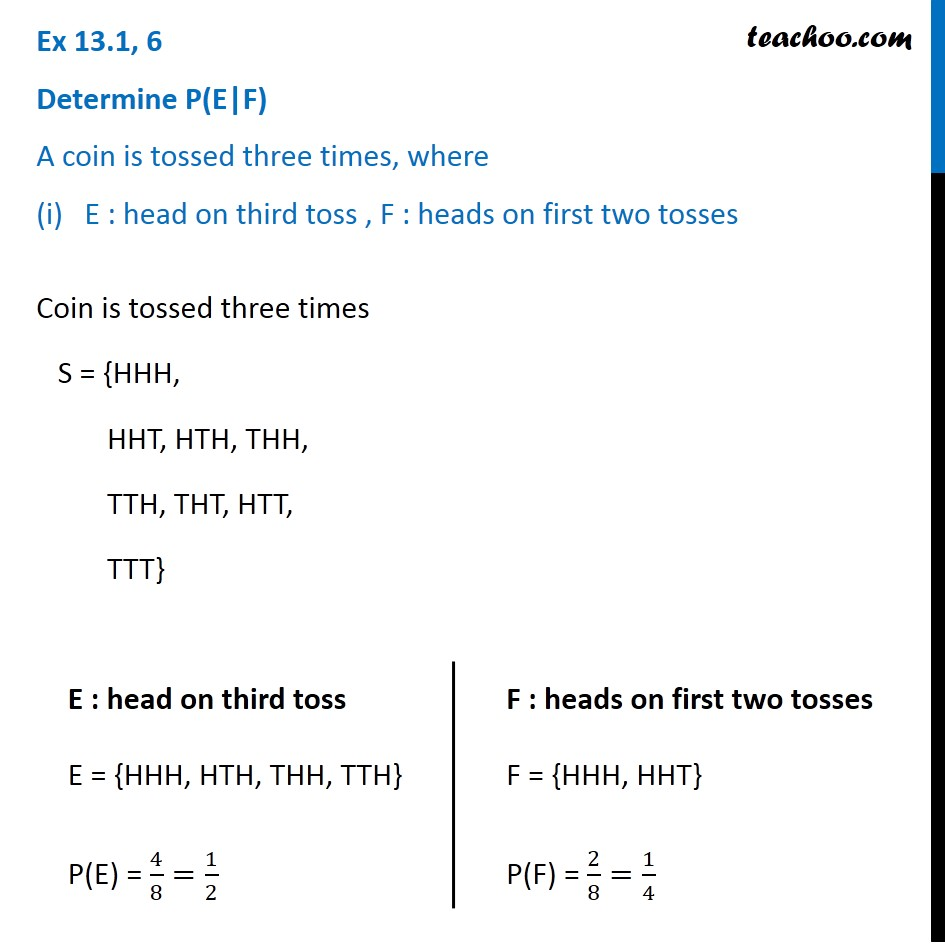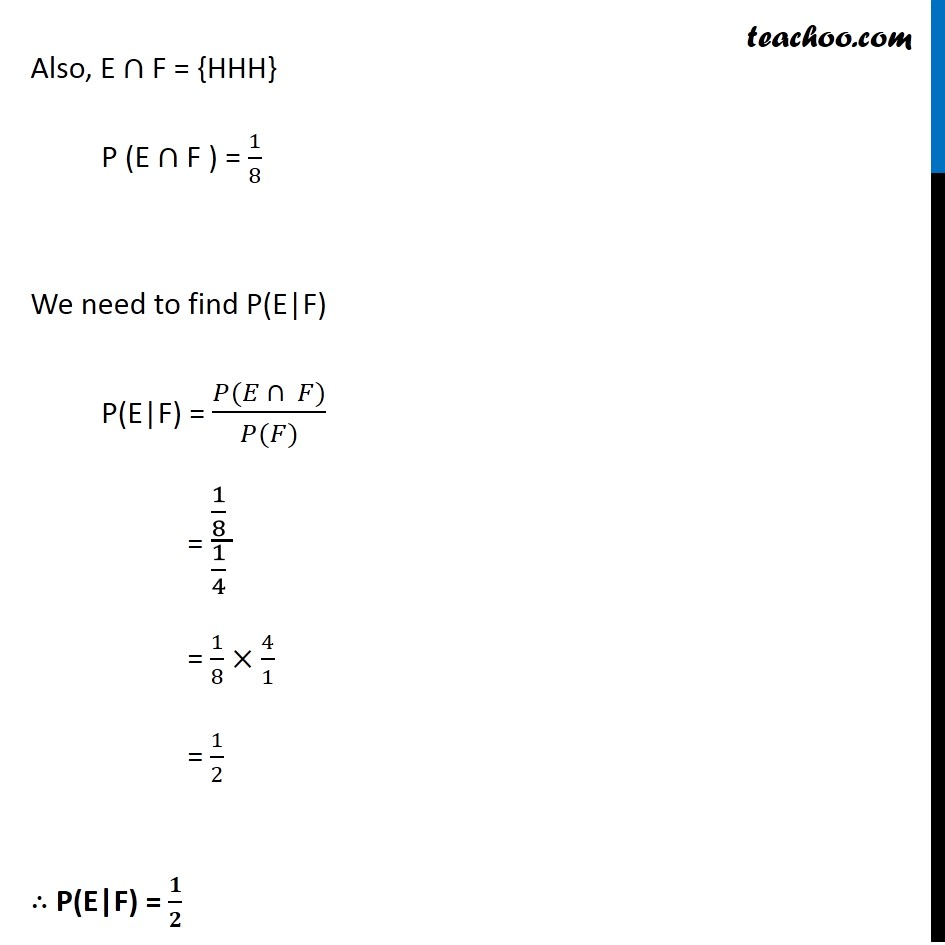Conditional Probability - Statement

Chapter 13 Class 12 Probability
Concept wiseLearn in your speed, with individual attention - Teachoo Maths 1-on-1 Class

### Transcript

Ex 13.1, 6 ﻿Determine P(E|F) A coin is tossed three times, where (i) E : head on third toss , F : heads on first two tossesCoin is tossed three times S = {HHH, HHT, HTH, THH, TTH, THT, HTT, TTT} E : head on third toss E = {HHH, HTH, THH, TTH} P(E) = 4/8=1/2 F : heads on first two tosses F = {HHH, HHT} P(F) = 2/8=1/4 Also, E ∩ F = {HHH} P (E ∩ F ) = 1/8 We need to find P(E|F) P(E|F) = (𝑃(𝐸 ∩ 𝐹))/(𝑃(𝐹)) = (1/8 )/(1/4 ) = 1/8×4/1 = 1/2 ∴ P(E|F) = 𝟏/𝟐